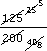Science, Maths & Technology

### Become an OU studentRatio, proportion and percentages

Start this free course now. Just create an account and sign in. Enrol and complete the course for a free statement of participation or digital badge if available.

# 1.4 Converting ratios from fractions to decimals

Although ratios are often given as fractions, they can also be expressed as decimals. You need to deal with a mixture of fractions and decimals, and to compare ratios given in either form, so you need to be able to convert between the two forms.

## Example 4

The ratio of the circumference of a circle to its diameter is a constant denoted by(the Greek letter p) pronounced pi, it has been approximated by a number of different fractions. One such fraction is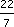, another is 355/113. How do these compare with the decimal value from a calculator of 3.141592 654?

If you have a calculator handy then you could key in 22 ÷ 7 to convert to a decimal. However, if not, you might use long division or an informal method of division. Either way you should get 3.1428 …. Soagrees to 3 significant figures.

You will probably find it easier to use a calculator for dividing 355 by 113. The result is 3.14159292, which agrees to 7 significant figures.

Sometimes you are given a ratio as a decimal, but might find it easier to use and/or remember as a fraction.

## Example 5

Suppose you had been told that the ratio between a distance measured in miles and the same distance measured in kilometres is about 0.625. Convert this to a fraction.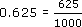This fraction is an adequate answer, or you can divide top and bottom by common factors to reach a simpler equivalent fraction.

Divide top and bottom by 5 to get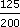.

Divide top and bottom by 5 again to get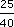.

Divide top and bottom by 5 again to get.

So the fraction is, which is the fraction used earlier.

An alternative method is do this in one line cancelling